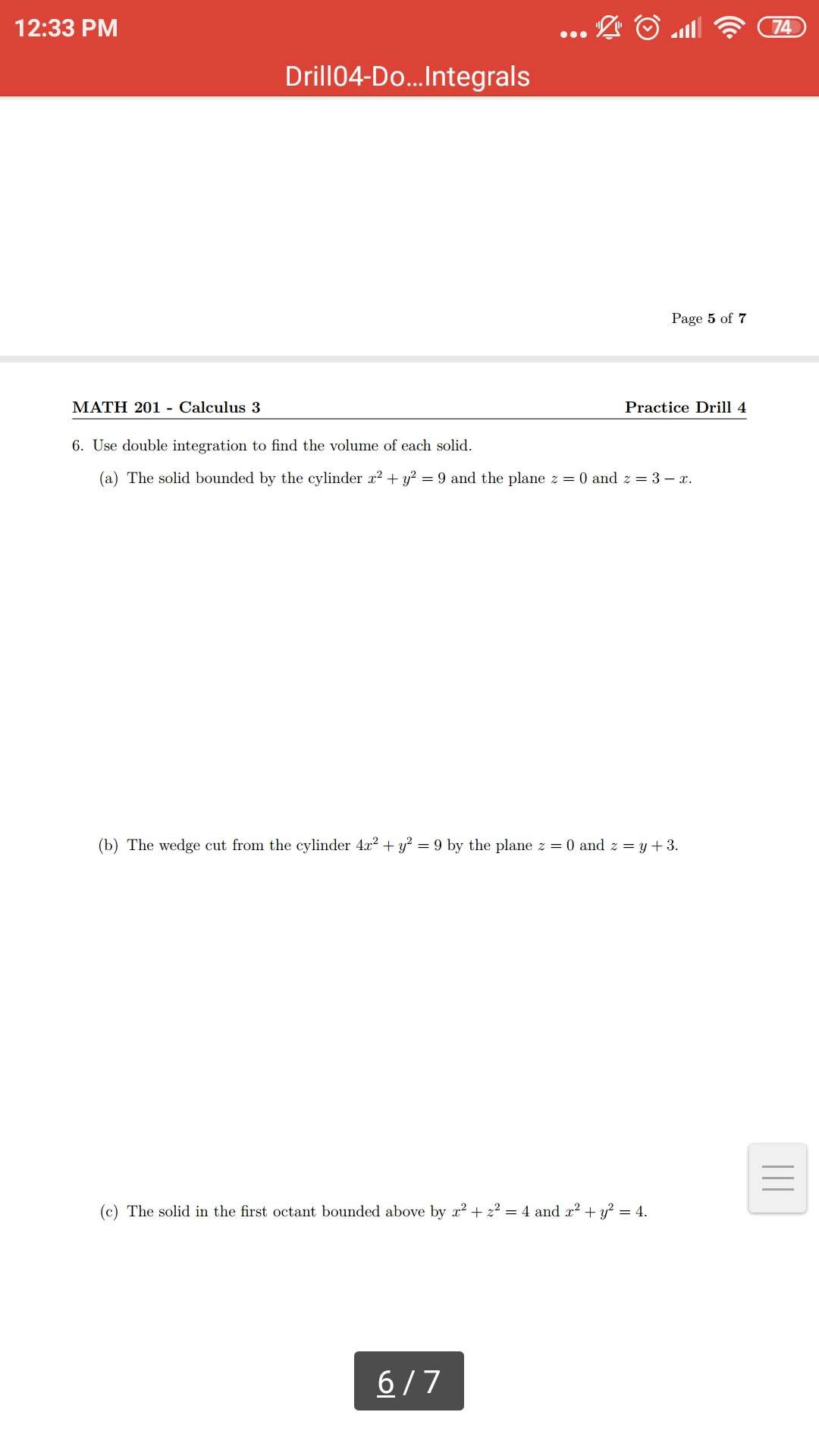# 7412:33 PMDrill04-Do...IntegralsPage 5 of 7MATH 201 - Calculus 3Practice Drill 46. Use double integration to find the volume of each solid(a) The solid bounded by the cylinder x2 +y2 = 9 and the plane z = 0 and z = 3 - x(b) The wedge cut from the cylinder 4x2 +y2 = 9 by the plane z = 0 and z = y +3(c) The solid in the first octant bounded above by x222 = 4 and x2-+y2 = 46/7

Question
14 views

Use double integration to find the volume of the solid

Question:

The wedge cut from the cylinder 4x2+y2=9 by the plane z=0 and z=y+3help_outlineImage Transcriptionclose74 12:33 PM Drill04-Do...Integrals Page 5 of 7 MATH 201 - Calculus 3 Practice Drill 4 6. Use double integration to find the volume of each solid (a) The solid bounded by the cylinder x2 +y2 = 9 and the plane z = 0 and z = 3 - x (b) The wedge cut from the cylinder 4x2 +y2 = 9 by the plane z = 0 and z = y +3 (c) The solid in the first octant bounded above by x222 = 4 and x2-+y2 = 4 6/7 fullscreen
check_circle

Step 1
Step 2

### Want to see the full answer?

See Solution

#### Want to see this answer and more?

Solutions are written by subject experts who are available 24/7. Questions are typically answered within 1 hour.*

See Solution
*Response times may vary by subject and question.
Tagged in

### Calculus